# HBSE 9th Class Maths Solutions Chapter 7 Triangles Ex 7.5

Haryana State Board HBSE 9th Class Maths Solutions Chapter 7 Triangles Ex 7.5 Textbook Exercise Questions and Answers.

## Haryana Board 9th Class Maths Solutions Chapter 7 Triangles Exercise 7.5

Question 1.
ABC is a triangle. Locate a point in the interior of ΔABC which is equidistant from all the vertices of ΔABC.
Solution:
(i) Draw a triangle ΔABC.
(ii) Draw the perpendicular bisector PQ and RS on AB and BC respectively, intersecting each other at O.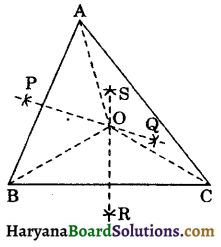(iii) Join OA, OB and OC. Here OA = OB = OC.
Hence, point O is the point which is equidistant from the vertices of ΔABC.Question 2.
In a triangle locate a point in its interior which is equidistant from all the sides of the triangle
Solution:
(i) Draw a triangle ΔABC.
(ii) Draw the bisectors BO and CO of ∠B and ∠C respectively, intersecting each other at O.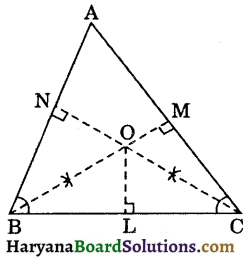(iii) Draw OL ⊥ BC, OM ⊥ CA and ON ⊥ AB, meeting BC at L. CA at M and AB at N respectively.
(iv) Here OL = OM = ON
Hence, point O is the point which is equidistant from the all sides of the triangle.

Question 3.
In a huge park, people are concentrated at three points (see figure 7.81).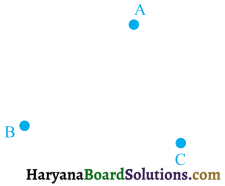A: Where there are different slides and swings for children.
B: Near which a man-made lake is situated.
C: Which is near to a large parking and exit. Where should an ice cream parlour be set up so that maximum number of persons can approach it?
[Hint: The parlour should be equidistant from A, B and C.]
Solution :
Join A to B and B to C. Parlour should be equidistant from A, B and C.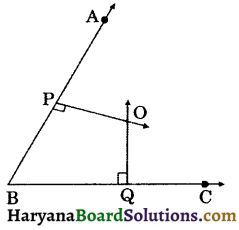Let PO and OQ be the perpendicular bisector of AB and BC respectively. They intersect at O. Since OP ⊥ bisector of AB. Therefore will equidistant from A and B.

Again, OQ ⊥ bisector of BC, therefore o will equidistant from B and C. Thus, O is the required point which is equidistant from A, B and C.Question 4.
Complete the hexagonal and star-shaped Rangolies (see figure 7.83 (i) and (ii)) by filling them with as many equilateral triangles of side 1 cm as you can. Count the number of triangles in each case. Which has more triangles?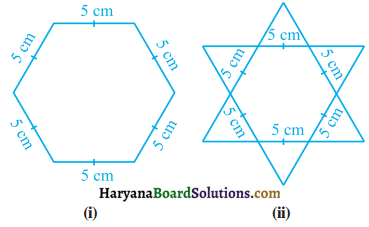Solution:
We divide the given hexagon into six equilateral triangles whose each side is 5 cm. Now take one such triangle and divide it into many equilateral of side 1 cm, as shown in the figure :
On counting number of triangles of side 1 cm each are :
Number of Δs in Ist row = 9
Number of Δs in Ilnd row = 7
Number of Δs in Illrd row = 5
Number of Δs in IVth row = 3
Number of Δs in Vth row = 1
Total number of triangles = 25
∴ Total number of triangles in the hexagon = 6 × 25 = 150.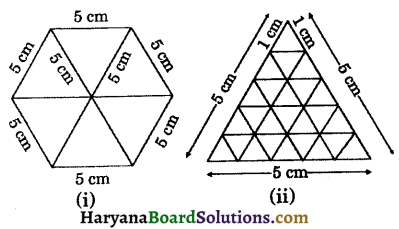(ii) Total number of triangles in figure 7.172
= 12 × 25
= 300.
Clearly, star-shaped Rangoli has more triangles.